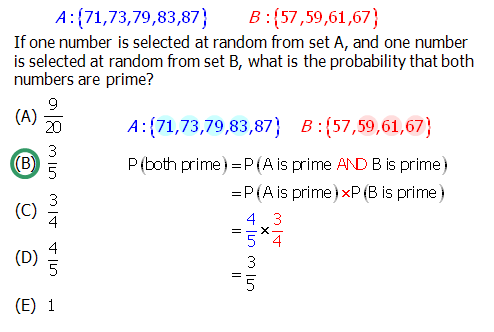# Divisibility Rules and Shortcuts

Actually, on the new IR questions coming this summer, there will be an onscreen calculator, but for the ordinary Quantitative questions, for the foreseeable future, you will be doing those without a calculator of any sort.

Consider the following question:

S = {71, 73, 75, 77, 79, 81, 83, 85, 87, 89}

How many numbers in S are prime?

## The Easy Rules

First of all, it’s probably obvious that all even numbers are divisible by 2. Furthermore, 2 is the only even prime number: all other positive even integers are divisible by 2, so all other prime numbers are odd.

A number that ends with a 5 or a 0 is divisible by 5. A two digit number in which the first and last digits are the same is divisible by 11.

With 4, there’s an interesting pattern. Think of the one-digit numbers that are multiples of 4: those are 0, 4, and 8. If the last digit is 0, 4, or 8, and the tens digit is even, then the number is divisible by 4. If the last digit is even but not one of those — viz. 2 or 6, the one-digit even numbers that are not multiples of 4 – and the tens digit is odd, then the number is divisible by 4.

## The Pattern with 3

The rule for 3 is a bit different from the rules for other numbers. You simply add up the digits. If the sum of the digits is divisible by 3, than the original number is divisible by 3. For example, consider the number 285 — 2 + 8 + 5 = 15, which is divisible by 3, so that means 285 must be divisible by 3. Consider the number 2012 —- 2 + 0 + 1 + 2 = 5, which is not divisible by 3, so that means 2012 is not divisible by 3.

That pattern also works with 9 — if the sum of the digits is divisible by 9, then the original number is divisible by 9. For example, the next year that will be a multiple of 9 is 2016, because 2 + 0 + 1 + 6 = 9.

## Testing Prime Numbers

The QUANT occasionally will have questions that require you to figure out whether larger two-digit numbers are prime. It will not ask you to figure out whether numbers greater than 100 are prime. For numbers less than 100, two-digit numbers, it’s enough to check whether it divisible by any single digit prime number {2, 3, 5, 7}. Any two-digit number not divisible by one of those four must be prime.

Back to our set above:

S = {71, 73, 75, 77, 79, 81, 83, 85, 87, 89}

All odds, so no multiples of 2.The numbers 75 and 85 are obviously divisible by 5, so those are not prime.

S = {71, 73, __, 77, 79, 81, 83, __, 87, 89}

The number 77 = 7*11. Once we have that multiple of 7, we add and subtract 7 to find other multiples of 7: { . . . . 70, 77, 84, 91, . . . } We see that 77 is the only multiple of 7 on this list.

S = {71, 73, __, __, 79, 81, 83, __, 87, 89}

Now we need a multiple of 3. We notice that, with 81, 8+1=9, which is divisible by 3, so 81 must be divisible by 3. We add and subtract 3 repeatedly to get other multiples of three: { . . . 69, 72, 75, 78, 81, 84, 87, 90, . . .}. Just to double-check 87, we see 8+7=15, which is divisible by 3, so of course 87 is divisible by 3. We can eliminate

S = {71, 73, __, __, 79, __, 83, __, __, 89}

The remaining five numbers must be prime. Indeed, each one is a prime number.

Q1. A: {71,73,79,83,87} B:{57,59,61,67}

If one number is selected at random from set A, and one number is selected at random from set B, what is the probability that both numbers are prime?

A. 9/20

B. 3/5

C. 3/4

D. 4/5

E. 1